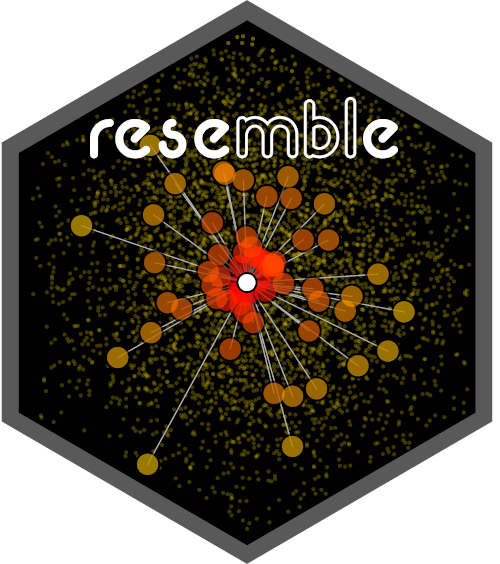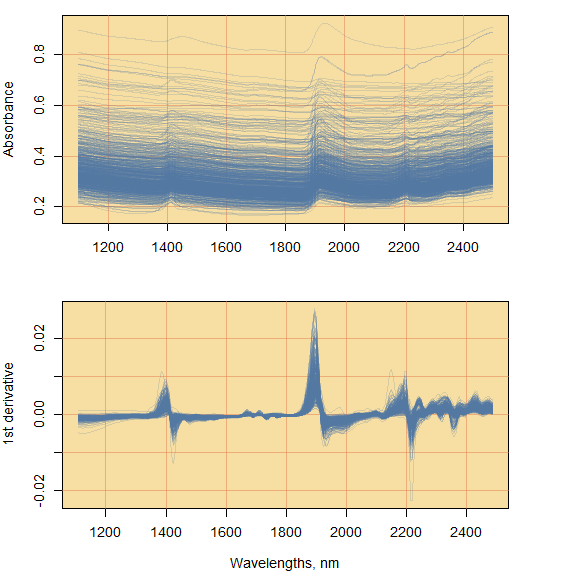Think Globally, Fit Locally# 1 Introduction

Modeling spectral data has garnered wide interest in the last four decades. Spectroscopy is the study of the spectral response of a matrix (e.g. soil, plant material, seeds, etc.) when it interacts with electromagnetic radiation. This spectral response directly or indirectly relates to a wide range of compositional characteristics (chemical, physical or biological) of the matrix. Therefore, it is possible to develop empirical models that can accurately quantify properties of different matrices. In this respect, quantitative spectroscopy techniques are usually fast, non-destructive and cost-efficient in comparison to conventional laboratory methods used in the analyses of such matrices. This has resulted in the development of comprehensive spectral databases for several agricultural products comprising large amounts of observations. The size of such databases increases de facto their complexity. To analyze large and complex spectral data, one must then resort to numerical and statistical tools and methods such as dimensionality reduction, and local spectroscopic modeling based on spectral dissimilarity concepts.

The aim of the resemble package is to provide tools to efficiently and accurately extract meaningful quantitative information from large and complex spectral databases. The core functionalities of the package include:

• dimensionality reduction
• computation of dissimilarity measures
• evaluation of dissimilarity matrices
• spectral neighbour search
• fitting and predicting local spectroscopic models

# 2 Citing the package

Simply type and you will get the info you need:

citation(package = "resemble")
##
## To cite resemble in publications use:
##
##   Ramirez-Lopez, L., and Stevens, A., and Viscarra Rossel, R., and
##   Lobsey, C., and Wadoux, A., and Breure, T. (2022). resemble:
##   Regression and similarity evaluation for memory-based learning in
##   spectral chemometrics. R package Vignette R package version 2.2.1.
##
## A BibTeX entry for LaTeX users is
##
##   @Manual{,
##     title = {resemble: Regression and similarity evaluation for memory-based learning in spectral chemometrics. },
##     author = {Leonardo Ramirez-Lopez and Antoine Stevens and Claudio Orellano and Raphael Viscarra Rossel and Zefang Shen and Craig Lobsey and Alex Wadoux},
##     publication = {R package Vignette},
##     year = {2022},
##     note = {R package version 2.2.1},
##     url = {https://CRAN.R-project.org/package=resemble},
##   }

# 3 Example dataset

This vignette uses the soil Near-Infrared (NIR) spectral dataset provided in the package prospectr package . The reason why we use this dataset is because soils are one of the most complex matrices analyzed with NIR spectroscopy. This spectral dataset/library was used in the challenge by Pierna and Dardenne (2008). The library contains NIR absorbance spectra of dried and sieved 825 soil observations/samples. These samples originate from agricultural fields collected from all over the Walloon region in Belgium. The data are in an R data.frame object which is organized as follows:

• Response variables:

• Nt (Total Nitrogen in g/kg of dry soil): a numerical variable (values are available for 645 samples and missing for 180 samples).

• Ciso (Carbon in g/100 g of dry soil): a numerical variable (values are available for 732 and missing for 93 samples).

• CEC (Cation Exchange Capacity in meq/100 g of dry soil): A numerical variable (values are available for 447 and missing for 378 samples).

• Predictor variables: the predictor variables are in a matrix embedded in the data frame, which can be accessed via NIRsoil$spc. These variables contain the NIR absorbance spectra of the samples recorded between the 1100 nm and 2498 nm of the electromagnetic spectrum at 2 nm interval. Each column name in the matrix of spectra represents a specific wavelength (in nm). • Set: a binary variable that indicates whether the samples belong to the training subset (represented by 1, 618 samples) or to the test subset (represented by 0, 207 samples). Load the necessary packages and data: library(resemble) library(prospectr) library(magrittr) The dataset can be loaded into R as follows: data(NIRsoil) dim(NIRsoil) str(NIRsoil) # 4 Spectra pre-processing This step aims at improving the signal quality of the spectra for quantitative analysis. In this respect, the following standard methods are applied using the package prospectr : 1. Resampling from a resolution of 2 nm to a resolution of 5 nm. 2. First derivative using Savitsky-Golay filtering . # obtain a numeric vector of the wavelengths at which spectra is recorded wavs <- NIRsoil$spc %>% colnames() %>% as.numeric()

# pre-process the spectra:
# - resample it to a resolution of 6 nm
# - use first order derivative
new_res <- 5
poly_order <- 1
window <- 5
diff_order <- 1

NIRsoil$spc_p <- NIRsoil$spc %>%
resample(wav = wavs, new.wav = seq(min(wavs), max(wavs), by = new_res)) %>%
savitzkyGolay(p = poly_order, w = window, m = diff_order)Figure 4.1: Raw spectral absorbance data (top) and first derivative of the absorbance spectra (bottom).

new_wavs <- as.matrix(as.numeric(colnames(NIRsoil$spc_p))) matplot(x = wavs, y = t(NIRsoil$spc),
xlab = "Wavelengths, nm",
ylab = "Absorbance",
type = "l", lty = 1, col = "#5177A133")

matplot(x = new_wavs, y = t(NIRsoil$spc_p), xlab = "Wavelengths, nm", ylab = "1st derivative", type = "l", lty = 1, col = "#5177A133") Both the raw absorbance spectra and the first derivative spectra are shown in Figure 4.1. The first derivative spectra represents the explanatory variables that will be used for all the examples throughout this document. For more explicit examples, the NIRsoil data is split into training and testing subsets: # training dataset training <- NIRsoil[NIRsoil$train == 1, ]
# testing dataset
testing  <- NIRsoil[NIRsoil$train == 0, ] In the resemble package we use the following notation (Ramirez-Lopez, Behrens, Schmidt, Stevens, et al. (2013)): • Training observations: • Xr stands for the matrix of predictor variables in the reference/training set (spectral data for calibration). • Yr stands for the response variable(s) in the reference/training set (dependent variable for calibration). In the context of this package, Yr is also referred as to “side information”, which is a variable or set of variables that are associated to the training observations that can also be used to support or guide optimization during modeling, but that not necessarily are part of the input of such models. For example, we will see in latter sections that Yr can be used in Principal Component Analysis to help on deciding how many components are optimal. • Testing observations: • Xu stands for the matrix of predictor variables in the unknown/test set (spectral data for validation/testing). • Yu stands for the response variable(s) in the unknown/test set (dependent variable for testing). # 5 Dimensionality reduction When conducting exploratory analysis of spectral data, we face the curse of dimensionality. It is such that we may be dealing with (using NIR spectra data as an example) hundreds to thousands of individual wavelengths for each spectrum. When one wants to find patterns in the data, spectral similarities and differences, or detect spectral outliers, it is necessary to reduce the dimension of the spectra while retaining important information. Principal Component (PC) analysis and Partial Least Squares (PLS) decomposition methods assume that the meaningful structure the data intrinsically lies on a lower dimensional space. Both methods attempt to find a projection matrix that projects the original variables onto a less complex subspaces (represented by new few variables). These new variables mimic the original variability across observations. These two methods can be considered as the standard ones for dimensionality reduction in many fields of spectroscopic analysis. The difference between PC and PLS is that in PC the objective is to find few new variables (which are orthogonal) that capture as much of the original data variance while in the latter the objective is to find few new variables that maximize their variance with respect to a set of one or more external variables (e.g. response variables or side information variables). In PC and PLS the input spectra ($$X$$, of $$n \times d$$ dimensions) is decomposed into two main matrices: a matrix of scores ($$T$$) and a matrix of ladings ($$P$$), so that: $X = T \: P + \varepsilon$ where the dimensions of $$T$$ and $$P$$ are $$n \times o$$ and $$o \times d$$, and where $$o$$ represents a given number of components being retained and $$\varepsilon$$ represents the reconstruction error. The maximum $$o$$ (number of components) that can be retrieved is limited to $$\textrm{min}(n-1, d)$$. One interesting property of $$P$$ is that it is equivalent to $$P^{-1}$$. This implies that when the PC decomposition is estimated for a given set of observations ($$X_{new}$$) the resulting $$P$$ matrix can be directly used to project new spectra onto the same principal component space by: $T_{new} = X_{new}\:P'$ ## 5.1 Methods In the resemble package, PC analysis and PLS decomposition are available through the ortho_projection() function which offers the following algorithms: • "pca": the standard method for PC analysis based on the singular value decomposition algorithm. • "pca.nipals": this algorithm uses the non-linear iterative partial least squares algorithm (NIPALS, H. Wold 1975) for the purpose of PC analysis. • "pls": Here, PLS decomposition also uses the NIPALS algorithm, but in this case it makes use of side information, which can be a variable or set of variables that are associated to the training observations and that are used to project the data. In this case, the variance between the projected variables and the side information variable(s) is maximized. The PC analysis of the training spectra can be executed as follows: # principal component (pc) analysis with the default # method (singular value decomposition) pca_tr <- ortho_projection(Xr = training$spc_p, method = "pca")

pca_tr

Plot the ortho_projection object:

plot(pca_tr, col = "#D42B08CC")
## Warning in par(opar): argument 1 does not name a graphical parameter